Next: Time-resolved spectra of G 226-29 Up: Modeling time-resolved spectra Previous: Introduction

# Time-resolved spectra and non-linear effects

There is more than one aim in our calculations. First, we want to be able to calculate amplitude spectra of oscillations. In Fig. 1 we show for l=1 to l=4 the normalized amplitude spectra (which are independent, for small amplitudes, of the often unknown inclination i; e.g., RKN, BFW). As one can see from Fig. 1, the slope varies strongly with l. By comparing the observed slope with the theoretical slope, one can therefore determine l (the slope also depends on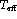and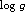, which may have to be constrained from other observations).Figure: Normalized amplitude spectra for l=1 (solid), l=2 (dashed), l=3 (dotted) and l=4 (dash-dotted) modes (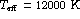,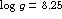,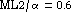). Around 1900 Å the phase of the l=4 mode changes by, causing the amplitude to go to zero

A second aim of our program is to calculate time-resolved spectra. This is shown in Fig. 2 for l=1, m=0 for a strong oscillation resulting in variations ofof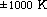. One can see that the spectra depend strongly on the inclination. Furthermore, one can see the effects of having a distribution ofat maximum and minimum instead of a uniformacross the WD surface.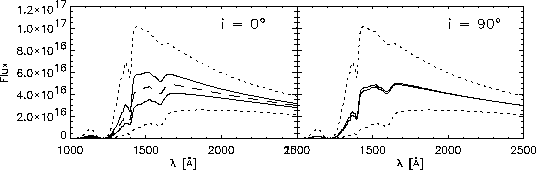Figure 2: UV-spectra at maximum and minimum (solid) for l=1, m=0 oscillations with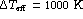for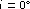(left) and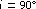(right). Also shown are the equilibrium spectrum (,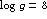,; dashed) and spectra at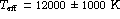(dotted)

A third aim is to properly take into account non-linear variations of I with linear, i.e., sinusoidal, variations of. For the above case we show in Fig. 3 the light curve for a filter located near the center of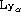and just outside this line. One can see that the light curve differs strongest from the underlying pure sinusoidal variation ofnear the center of the spectral line. This causes strong amplitudes at higher harmonics in the Fourier spectrum.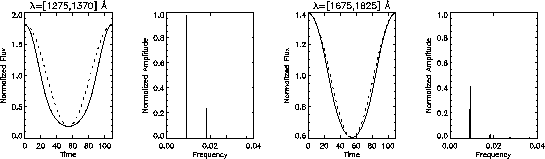Figure 3: Light curves and corresponding Fourier spectra for oscillations as in Fig. 2 withclose to the center of theline (left panels) and just outside theline (right panels). The underlying sinusoidal variation ofis overplotted (dotted)Next: Time-resolved spectra of G 226-29 Up: Modeling time-resolved spectra Previous: Introduction

Horst Vaeth
Thu Sep 26 10:49:14 MET DST 1996